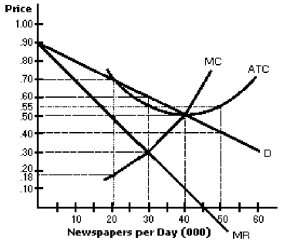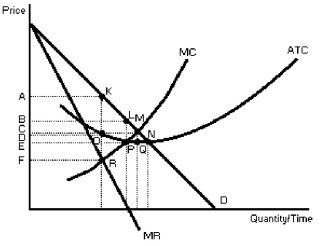/
/
/
71) In equilibrium, which of the following conditions common to
Not my Question
Flag Content

# Question : 71) In equilibrium, which of the following conditions common to : 1867572

71) In equilibrium, which of the following conditions is common to both unregulated monopoly and pure competition?

A) P = MR

B) AR = ATC

C) MR = MC

D) MC = P

72) The price elasticity of demand for a good at the output that maximizes a monopolist's profit

A) equals zero as long as the good has no close substitutes.

B) is always greater than one, because marginal revenue must be positive.

C) does not equal zero because there will always be some substitutes, however imperfect they may be.

D) does not equal zero because every good has at least one good substitute for it.

Figure 10-473) In Figure 10-4, the profit maximizing price-output combination for the monopolist is

A) 50 cents and an output of 40 000 newspapers per day.

B) 30 cents and an output of 30 000 newspapers per day.

C) 60 cents and an output of 30 000 newspapers per day.

D) 45 cents and an output of 45 000 newspapers per day.

74) In Figure 10-4, the profit maximizing price-output combination for the monopolist is ________

75) In Figure 10-4, the maximum profit the monopolist can make is

A) 0.

B) \$1 500 per day.

C) \$9 000 per day.

D) \$ 1 500 per day.

76) In Figure 10-4, the maximum profit the monopolist can make is ________

Figure 10-577) In Figure 10-5, the profit-maximizing price-output combination for the monopolist is shown at point

A) K.

B) R.

C) P.

D) N.

78) In Figure 10-5, the profit-maximizing price for the monopolist is

A) A.

B) B.

C) C.

D) D.

79) In Figure 10-5, when the monopolist maximizes profits, its profits are equal to the area given by

A) AKMC.

B) AKOD.

C) BLPE.

D) EPRF.

80

## Solution 5 (1 Ratings )

Solved
Economics 2 Days Ago 9 Views